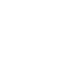# Apr 7 ACT & SAT Question of the Day

If you are reading this in an email you received from me, do not click the link to sat.collegeboard.org below. Use the link to my website that is farther down on the email. If you are seeing this in my blog, do the SAT Question of the Day by clicking on this link:

http://sat.collegeboard.org/practice/sat-question-of-the-day?src=R&questionId=20130407 (This link takes you to today’s question. If you use my archive, you will see the question related to my SAT explanation for that date.)

The answer is C.  The concept of “the difference of two squares” is on the SAT and ACT an awful lot.  That is, the area of one square (in this case, y) is subtracted from the area of another square (x).  You need to recognize the questions as “factoring” exercises and that the factors will always be (x+y) and (x-y).

There are two common questions that are asked about the difference of two squares.  Today’s question is the most common type.  The test writers will give you two values of the three that you can determine from using the binomial (x2y2) and its two factors: x+y and x-y.  In this case, they gave you the two factors are equal to 3 and 5 respectively and you simply had to multiply the two factors (3 and 5) to get the value of the binomial which is 15, Answer C.

Sometimes they will give you the value of the binomial and one of the factors and you’ll need to determine the value of the other factor.   For example, they could have given you x2y2=15 and x+y=3 and ask for the value of x-y.  Then, of course, it is a simple matter of dividing 15/3=5 to get the value of x-y.

The second question that pops up on the test that is related to this same concept is one that my students tell me that they haven’t been taught or that at least they don’t remember being taught.  In this case, the test writers may have asked you to solve for x and y.  It is very simple.  x is the average of the value of the two factors (x+y and x-y) or 4 in this sample question.   (Notice that the average is half way between the two factors; 4 is half way between 3 and 5.)  Then you can easily solve for y by substituting 4 into either factor; in this example, it is 1.

Today’s question is an example of the many “flags” or “signals” you can use on the test to help raise your score.  For this question, you should recognize immediately you are playing with the difference of two squares and you can do the simple math manipulations that I described above.  Some other flags are “ratios,” in which case the key to answering the question is the sum of the parts.  For “circle” questions, the key is the radius.  For “independent events,” multiply the separate values.  For “mutually exclusive events,” their sum has to be 1.  Learning to recognize “flags” and their “keys” will make you much faster at doing the test questions and consequently will raise your score.

Let’s see what the ACT folks have on their minds this morning.

http://www.act.org/qotd/ (The ACT staff does not put a date on their questions so if you click on an archived blog, you’ll get today’s question and the old explanation. Sorry. The SAT staff has dated their questions; so, the archive is helpful. The ACT folks simply don’t do that.)

The answer is J.  This is a common mistake that is tested on both the ACT and the SAT.  You were pretty young when your teachers started explaining the differences of “they’re,” “their,” and “there.”  If you see one of the three words underlined on a grammar part of the test (the “flag” or “signal”), the key will to simply verify that the proper spelling has been used for the word that is underlined.  In this question, “they’re” is the the contraction for “they are” and it doesn’t make sense.  The sentence is about Fokker being in some particular location; so, we need “there.”  That’s easy enough.  However, don’t fall for the trap answer “there.”  You also have to keep the rest of the underlined words without changing them, Answer J.  If you just fix the mistake by changing “they’re” to “there” and get answer H, you’ve made a silly mistake.  Be careful.

I hope you have a great day.  Make it even better by thanking somebody for being your friend.

Wizard